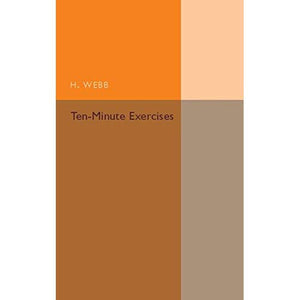># Ten-Minute Exercises

• £13.29
• Save £12

H. Webb
Cambridge University Press, 3/3/2016
EAN 9781316601822, ISBN10: 131660182X

Paperback, 200 pages, 20.3 x 12.8 x 1.3 cm
Language: English

Originally published in 1938, this book provides a series of exercises in arithmetic intended to take pupils ten minutes to complete. The text was created to train pupils in speed and accuracy in the fundamentals of arithmetic, avoiding unnecessary written work. This book will be of value to anyone with an interest in arithmetic, mathematics and the history of education.

Introduction
Extracts from the Board of Education Handbook of Suggestions for the Teaching of Mathematics, 1937
Part I
1. Miscellaneous exercises
2. Football league tables
4. Multiplication and division
5. Reduction
6. Revision exercises
7. Short methods in multiplication and division
8. Costs of dozens
9. Costs in various quantities
10. Costs of scores and grosses
11. Miscellaneous exercises
12. Bills and change
13. Perimeters and areas of rectangles
14. Progress tests
15. Prime factors and L.C.M.
16. Fractions
17. Fractional qualities
18. Miscellaneous exercises
19. Decimals
20. Decimal qualities
21. Miscellaneous exercises
22. Progress tests
23. Averages and timetables
24. Percentages
25. Proportion
26. Mensuration and algebra
27. Sales' reductions
28. Progress and final tests
Part II
29. Miscellaneous exercises
30. Addition, subtraction, multiplication and division
31. Reduction
32. Short methods in multiplication and division
33. Costs of dozens
34. Costs in various quantities
35. Costs of scores and grosses
36. Bills and change
37. Perimeters and areas of rectangles
38. Squares and square roots
39. Arithmetic in daily life
40. Progress tests
41. Fractions and fractional quantities
42. Decimals and decimal quantities
43. Miscellaneous exercises
44. Averages, approximations and rough estimates
45. Time tables
46. Arithmetic in daily life
47. Triangles, parallelograms, circles and polygons
48. Revision tests
49. Progress tests
50. Percentages
51. Profit and loss, and simple interest
52. Commission and discount
53. Direct proportion
54. Inverse proportion
55. Algebra
56. Arithmetic in daily life
57. Progress tests and final tests
Part III
58. Miscellaneous exercises
59. Revision tests in the four roles
60. Reduction. Short methods
61. Costs of dozens, scores and grosses
62. Miscellaneous exercises
63. Square roots and areas. Circles
64. Arithmetic in daily life
65. Progress tests
66. Fractions and fractional qualities
67. Decimals and decimal qualities
68. Miscellaneous exercises
69. Volumes
70. More short methods and costs
71. Time sheets
72. Arithmetic in daily life
73. Progress tests
74. Percentages
75. Profit and loss, and simple interest
76. Commission and discount
77. Sales' reductions
78. Proportion - direct, inverse and compound
79. Arithmetic in daily life
80. Algebra
81. Revision tests in arithmetic
82. Revision tests in mensuration
83. Revision tests in algebra
84. Progress tests and final tests.